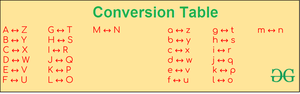GeeksforGeeks App
Open AppBrowser
Continue

# How To Encode And Decode A Message using Python?

Encryption is the process of converting a normal message (plain text) into a meaningless message (Ciphertext). Whereas, Decryption is the process of converting a meaningless message (Cipher text) into its original form (Plain text). In this article, we will take forward the idea of encryption and decryption and draft a python program.

In this article, we will be given a single-line message as input it is either encoded or decoded as per requirement and the resultant message is printed as output. Here, the conversion has been done by replacing A to Z, B to Y, … Z to A. The case of the characters, numbers, spaces, and special characters present in the message is being kept unchanged.Sample Example 1:

Encryption

```Input : Hello World
Output : Svool Dliow```

Explanation: (Reference- conversion table)

• H is replaced with S
• e is replaced with v
• l is replaced with o
• W is replaced with D
• r is replaced with i

Decryption

```Input : Svool Dliow
Output : Hello World ```

Explanation: (Reference- conversion table)

• S is replaced with H
• v is replaced with e
• o is replaced with l
• D is replaced with W
• i is replaced with r

Sample Example 2:

Encryption

```Input : GeeksForGeeks
Output : TvvphUliTvvph```

Explanation: (Reference- conversion table)

• G is replaced with T
• e is replaced with v
• k is replaced with p
• s is replaced with h
• F is replaced with U
• o is replaced with l
• r is replaced with i

Decryption

```Input : TvvphUliTvvph
Output : GeeksForGeeks```

Explanation: (Reference- conversion table)

• T is replaced with G
• v is replaced with e
• p is replaced with k
• h is replaced with s
• U is replaced with F
• l is replaced with o
• i is replaced with r

Below is the implementation.

## Python3

 `# Taking input from user``data ``=` `'Welcome to GeeksForGeeks...'`  `# conversion Chart``conversion_code ``=` `{` `    ``# Uppercase Alphabets``    ``'A'``: ``'Z'``, ``'B'``: ``'Y'``, ``'C'``: ``'X'``, ``'D'``: ``'W'``, ``'E'``: ``'V'``, ``'F'``: ``'U'``,``    ``'G'``: ``'T'``, ``'H'``: ``'S'``, ``'I'``: ``'R'``, ``'J'``: ``'Q'``, ``'K'``: ``'P'``, ``'L'``: ``'O'``,``    ``'M'``: ``'N'``, ``'N'``: ``'M'``, ``'O'``: ``'L'``, ``'P'``: ``'K'``, ``'Q'``: ``'J'``, ``'R'``: ``'I'``,``    ``'S'``: ``'H'``, ``'T'``: ``'G'``, ``'U'``: ``'F'``, ``'V'``: ``'E'``, ``'W'``: ``'D'``, ``'X'``: ``'C'``,``    ``'Y'``: ``'B'``, ``'Z'``: ``'A'``,` `    ``# Lowercase Alphabets``    ``'a'``: ``'z'``, ``'b'``: ``'y'``, ``'c'``: ``'x'``, ``'d'``: ``'w'``, ``'e'``: ``'v'``, ``'f'``: ``'u'``,``    ``'g'``: ``'t'``, ``'h'``: ``'s'``, ``'i'``: ``'r'``, ``'j'``: ``'q'``, ``'k'``: ``'p'``, ``'l'``: ``'o'``,``    ``'m'``: ``'n'``, ``'n'``: ``'m'``, ``'o'``: ``'l'``, ``'p'``: ``'k'``, ``'q'``: ``'j'``, ``'r'``: ``'i'``,``    ``'s'``: ``'h'``, ``'t'``: ``'g'``, ``'u'``: ``'F'``, ``'v'``: ``'e'``, ``'w'``: ``'d'``, ``'x'``: ``'c'``,``    ``'y'``: ``'b'``, ``'z'``: ``'a'``}` `# Creating converted output``converted_data ``=` `""`  `for` `i ``in` `range``(``0``, ``len``(data)):``    ``if` `data[i] ``in` `conversion_code.keys():``        ``converted_data ``+``=` `conversion_code[data[i]]``    ``else``:``        ``converted_data ``+``=` `data[i]` `# Printing converted output``print``(converted_data)`

Output

`Dvoxlnv gl TvvphUliTvvph...`

Approach: Using strings and index() method

## Python3

 `# Taking input from user``data ``=` `'Welcome to GeeksForGeeks...'``# conversion Chart``la``=``"abcdefghijklmnopqrstuvwxyz"``ua``=``"ABCDEFGHIJKLMNOPQRSTUVWXYZ"``lra``=``la[::``-``1``]``ura``=``ua[::``-``1``]``# Creating converted output``converted_data ``=` `""`  `for` `i ``in` `range``(``0``, ``len``(data)):``    ``if` `data[i] ``in` `la:``        ``converted_data``+``=``lra[la.index(data[i])]``    ``elif` `data[i] ``in` `ua:``        ``converted_data``+``=``ura[ua.index(data[i])]``    ``else``:``        ``converted_data``+``=``" "``# Printing converted output``print``(converted_data)`

Output

`Dvoxlnv gl TvvphUliTvvph   `

## Python3

 `# Fn to encrypt the message``def` `encrypt(message):``    ``conversion_table ``=` `str``.maketrans(``"abcdefghijklmnopqrstuvwxyzABCDEFGHIJKLMNOPQRSTUVWXYZ"``, ``"zyxwvutsrqponmlkjihgfedcbaZYXWVUTSRQPONMLKJIHGFEDCBA"``)``    ``return` `message.translate(conversion_table)`` ` `# Initializing the innput``message ``=` `"Hello World"` `# Calling Function``encrypted_message ``=` `encrypt(message)` `# print the encrypted messages``print``(encrypted_message)`

Output

`Svool Dliow`

My Personal Notes arrow_drop_up